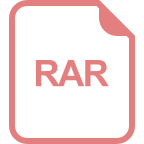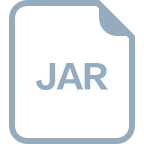# dbscan聚类算法python代码

### 回答1： DBSCAN是一种密度聚类算法，可以通过Python代码实现。以下是一个使用Python实现DBSCAN算法的示例代码： ```python from sklearn.cluster import DBSCAN from sklearn import datasets import matplotlib.pyplot as plt # 导入数据集 iris = datasets.load_iris() X = iris.data # 构建DBSCAN聚类模型 dbscan = DBSCAN(eps=0.4, min_samples=5) dbscan.fit(X) # 打印聚类标签 print("聚类标签: ", dbscan.labels_) # 绘制聚类结果 colors = ['b', 'g', 'r', 'c', 'm', 'y', 'k'] for i in range(len(X)): plt.scatter(X[i], X[i], c=colors[dbscan.labels_[i]]) plt.show() ``` 在这个示例中，我们使用Scikit-learn库中的DBSCAN模块进行聚类。首先，我们从Scikit-learn库中导入DBSCAN和数据集。然后，我们设置聚类模型的超参数，包括eps和min_samples。接下来，我们使用模型拟合数据，并打印每个点的聚类标签。最后，我们使用Matplotlib库绘制数据点和聚类结果。 ### 回答2： DBSCAN（Density-Based Spatial Clustering of Applications with Noise）是一种基于密度的聚类算法，可以自动发现任意形状的聚类。下面是DBSCAN聚类算法的Python代码实现： ```python import numpy as np from sklearn.cluster import DBSCAN from sklearn.datasets import make_moons from sklearn.preprocessing import StandardScaler import matplotlib.pyplot as plt # 生成一个月亮形状的数据集 X, y = make_moons(n_samples=200, noise=0.05, random_state=0) # 数据标准化 X = StandardScaler().fit_transform(X) # 使用DBSCAN进行聚类 dbscan = DBSCAN(eps=0.3, min_samples=5) labels = dbscan.fit_predict(X) # 可视化聚类结果 unique_labels = np.unique(labels) markers = ['o', 's', '^', 'D', 'x', 'v', 'p', '*', 'h', '+'] colors = plt.cm.Spectral(np.linspace(0, 1, len(unique_labels))) for (label, color) in zip(unique_labels, colors): class_member_mask = (labels == label) xy = X[class_member_mask] plt.scatter(xy[:, 0], xy[:, 1], marker=markers[label % len(markers)], color=color) plt.title('DBSCAN Clustering') plt.xlabel('Feature 1') plt.ylabel('Feature 2') plt.show() ``` 上述代码首先生成了一个月亮形状的数据集，然后对数据进行标准化处理。接下来使用DBSCAN算法进行聚类，其中`eps`参数定义了邻域半径的大小，`min_samples`参数定义了一个样本点的邻域内所需的最小样本数。最后使用Matplotlib库将聚类结果可视化出来。可以看到，代码中使用不同的标记和颜色来表示不同的聚类簇。 ### 回答3： DBSCAN（Density-Based Spatial Clustering of Applications with Noise）是一种基于密度的聚类算法，它通过将样本空间划分为具有足够密度的区域来进行聚类。以下是一个用Python实现的简单DBSCAN聚类算法的代码示例： ```python import numpy as np from sklearn.cluster import DBSCAN # 创建一个样本数据集 X = np.array([[1, 2], [1.5, 1.8], [5, 8], [8, 8], [1, 0.6], [9, 11]]) # 实例化DBSCAN对象，设置eps和min_samples参数 dbscan = DBSCAN(eps=0.3, min_samples=2) # 聚类 dbscan.fit(X) # 打印每个样本的标签 print(dbscan.labels_) ``` 上述代码中，我们首先导入了所需的库，然后创建了一个样本数据集X。接下来，我们使用`DBSCAN`类创建了一个DBSCAN对象，并设置了`eps`参数为0.3，`min_samples`参数为2。然后，我们使用`fit`方法对样本数据进行聚类。最后，我们打印出每个样本的标签，其中正数表示聚类的簇标签，-1表示噪音点。 当我们运行上述代码时，将会得到一个数组，其中包含每个样本的聚类标签。例如，[0 0 1 1 -1 2]表示前两个样本属于第一个簇，第三和第四个样本属于第二个簇，第五个样本被视为噪音点，第六个样本属于第三个簇。 当然，这个简单的例子只是DBSCAN算法的基本示例。实际中，我们可以根据具体的需求调整`eps`和`min_samples`参数的值，并使用其他可用的方法和函数来进一步分析和可视化聚类结果。

## 相关推荐